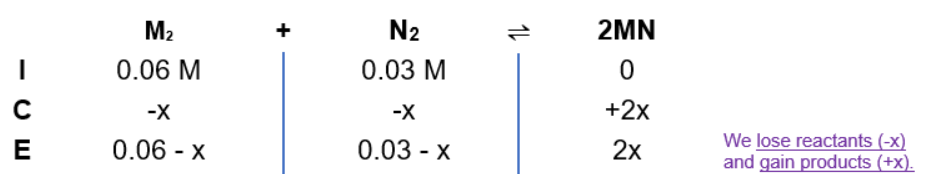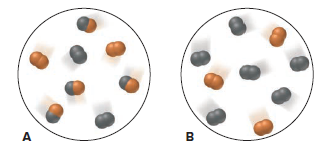# Problem: For the reaction M2 + N2 ⇌ 2MN, scene A represents the mixture at equilibrium, with M black and N orange. If each molecule represents 0.10 mol and the volume is 1.0 L, how many moles of each substance will be present in scene B when that mixture reaches equilibrium?

###### FREE Expert Solution

Equilibrium reaction: M2 + N2 ⇌ 2MN

When dealing with equilibrium and Kc:

Kc → equilibrium units are in molarity
Kc is an equilibrium expression:

$\overline{){{\mathbf{K}}}_{{\mathbf{c}}}{\mathbf{=}}\frac{\mathbf{products}}{\mathbf{reactants}}{\mathbf{=}}\frac{{\left[\mathrm{MN}\right]}^{\mathbf{2}}}{\left[{M}_{2}\right]\left[{N}_{2}\right]}}$

Solve for Kc using diagram A:

${\mathbf{K}}_{\mathbf{c}}\mathbf{=}\frac{{\left[\mathbf{0}\mathbf{.}\mathbf{04}\right]}^{\mathbf{2}}}{\left[\mathbf{0}\mathbf{.}\mathbf{02}\right]\left[\mathbf{0}\mathbf{.}\mathbf{02}\right]}\phantom{\rule{0ex}{0ex}}{\mathbf{K}}_{\mathbf{c}}\mathbf{=}\frac{\mathbf{1}\mathbf{.}\mathbf{6}\mathbf{×}{\mathbf{10}}^{\mathbf{-}\mathbf{3}}}{\mathbf{4}\mathbf{.}\mathbf{0}\mathbf{×}{\mathbf{10}}^{\mathbf{-}\mathbf{4}}}$

Kc = 4.0

ICE Chart for diagram B:99% (221 ratings)###### Problem Details

For the reaction M2 + N2 ⇌ 2MN, scene A represents the mixture at equilibrium, with M black and N orange. If each molecule represents 0.10 mol and the volume is 1.0 L, how many moles of each substance will be present in scene B when that mixture reaches equilibrium?